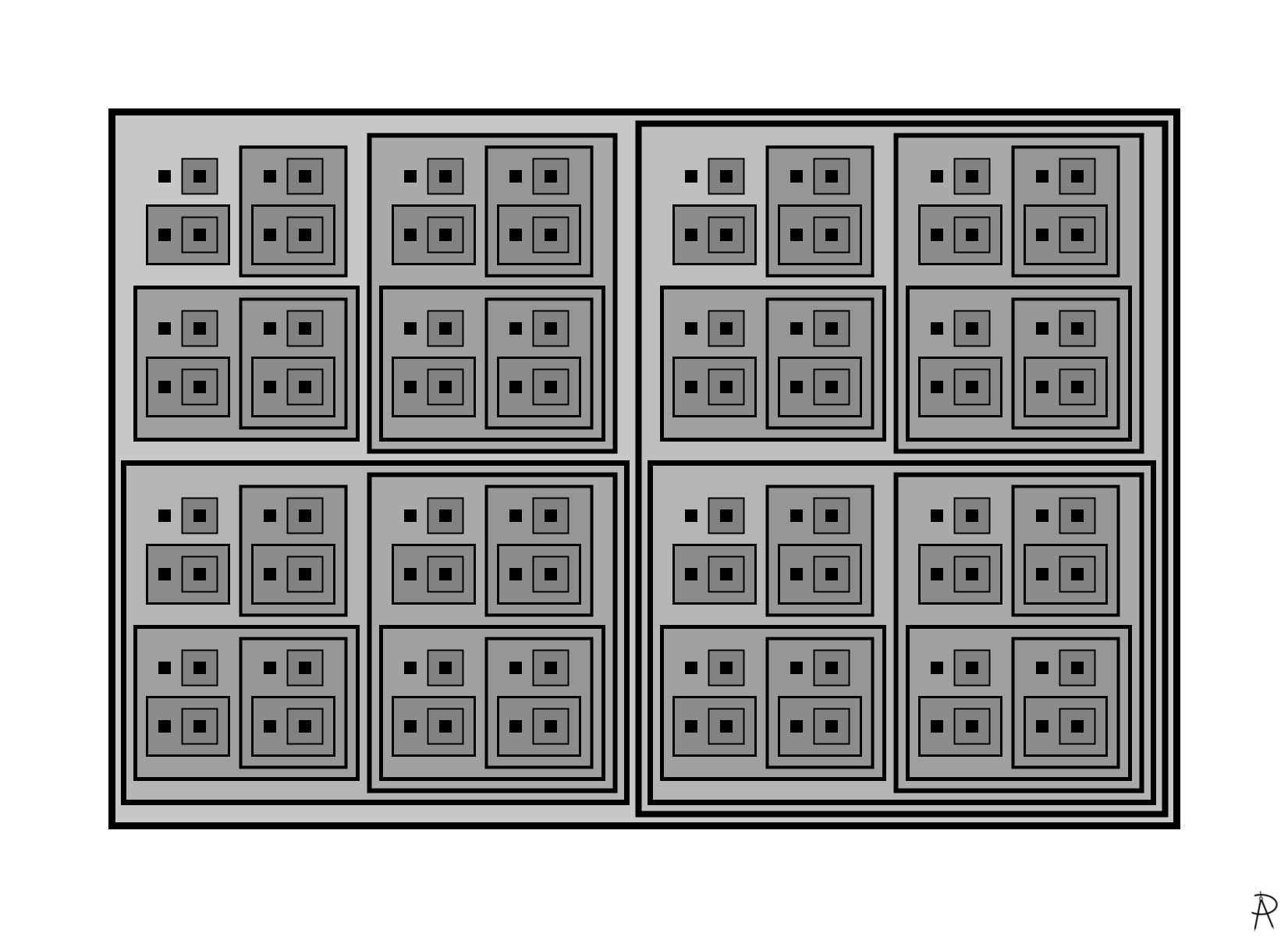Ordinal Eight Digital Print 2021

The number eight shown as a set-theoretic finite ordinal number using the construction of the non-negative integers described by John von Neumann around 1923.

### Background and Inspiration

How does one define the concept of number? What is the meaning of a number such as eight? One way to do this is from the perspective of a set.

In this set-theoretic finite ordinal number representation, zero is represented by the empty set: $$\{\}$$ or $$\emptyset$$. Given $$0 = \{\emptyset\}$$, we can define the integers recursively with the use of a successor function, $$s(n)$$, defined as follows: $s(n) = n + 1 = n \cup \{n\}.$ One, the successor of 0, is the set containing zero (the empty set): $$\{\{\}\}$$ or $$\{\emptyset\}$$. Two, the successor of 1, is the set containing zero and one: $$\{ \{\}, \{\{\}\}\}$$ or $$\{\emptyset, \{\emptyset\}\}$$. In general, the number $$n$$ is the successor of $$n-1$$ and the set containing the numbers $$0$$ through $$n-1$$: $$\{0, 1, 2, \ldots, n-1\}$$. In this piece, the empty set $$\emptyset$$ is depicted as a black square and sets are depicted by rectangles.

An interesting property of this construction is that $$a < b$$ can be defined using set operations. Let $$a$$ and $$b$$ be two numbers and $$A$$ and $$B$$ be the two sets representing them, respectively, then $$a < b$$ if and only if $$A \subset B$$.

You can see this pattern by examining the the first five integers expressed in set notation: \begin{aligned} 0 &= \emptyset\\ 1 &= \{\emptyset\}\\ &= \{0\}\\ 2 &= \{\emptyset,\ \{\emptyset\}\}\\ &= \{0, 1\}\\ 3 &= \{\emptyset,\ \{\emptyset\},\ \{\emptyset,\{\emptyset\}\}\}\\ &= \{0, 1, 2\}\\ 4 &= \{\emptyset,\ \{\emptyset\},\ \{\emptyset,\{\emptyset\}\},\ \{\emptyset,\{\emptyset\},\{\emptyset,\{\emptyset\}\}\}\}\\ &= \{0, 1, 2, 3\}\\ 5 &= \{\emptyset,\{\emptyset\},\{\emptyset,\{\emptyset\}\},\{\emptyset,\{\emptyset\},\{\emptyset,\{\emptyset\}\}\},\{\emptyset,\{\emptyset\},\{\emptyset,\{\emptyset\}\},\{\emptyset,\{\emptyset\},\{\emptyset,\{\emptyset\}\}\}\}\}\\ &= \{0, 1, 2, 3, 4\}\\ \end{aligned}

Furthermore, the number of elements of each set (its cardinality) is just the number itself. For example, the set representing 8 has 8 elements.